##### Circuit Analysis For Dummies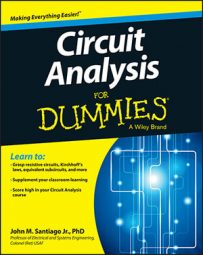Capacitors store energy for later use. The instantaneous power of a capacitor is the product of its instantaneous voltage and instantaneous current. To find the instantaneous power of the capacitor, you need the following power definition, which applies to any device: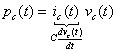The subscript C denotes a capacitance device (surprise!). Substituting the current for a capacitor into this equation gives you the following: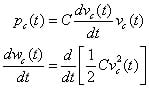Assuming zero initial voltage, the energy wC(t) stored per unit time is the power. Integrating that equation gives you the energy stored in a capacitor: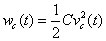The energy equation implies that the energy stored in a capacitor is always positive. The capacitor absorbs power from a circuit when storing energy. The capacitor releases the stored energy when delivering energy to the circuit.

For a numerical example, look at the top-left diagram shown here, which shows how the voltage changes across a 0.5-μF capacitor. Try calculating the capacitor’s energy and power.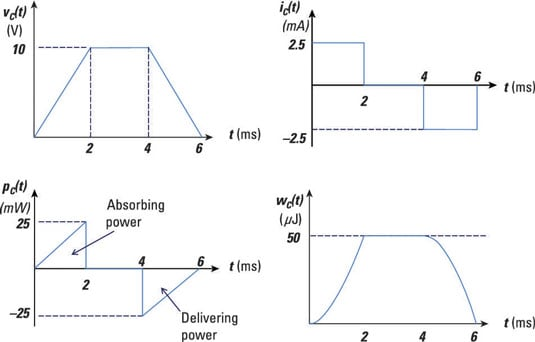The slope of the voltage change (time derivative) is the amount of current flowing through the capacitor. Because the slope is constant, the current through the capacitor is constant for the given slopes. For this example, you calculate the slope for each time interval in the graph as follows: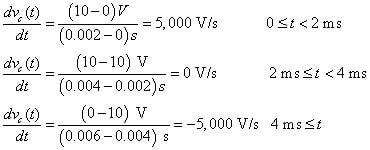Multiply the slopes by the capacitance (in farads) to get the capacitor current during each interval. The capacitance is 0.5 μF, or 0.5 × 10–6 F, so here are the currents: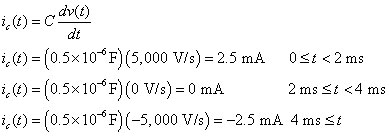You see the graph of the calculated currents in the top-right diagram shown here.You find the power by multiplying the current and voltage, resulting in the bottom-left graph shown here. Finally, you can find the energy by calculating (½)C[vC(t)]2. When you do this, you get the bottom-right graph shown here. Here, the capacitor’s energy increases when it’s absorbing power and decreases when it’s delivering power.